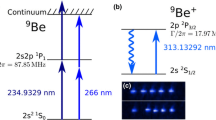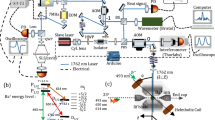# All-solid-state continuous-wave laser systems for ionization, cooling and quantum state manipulation of beryllium ions

## Abstract

We describe laser systems for photoionization, Doppler cooling, and quantum state manipulation of beryllium ions. For photoionization of neutral beryllium, we have developed a continuous-wave 235 nm source obtained by two stages of frequency doubling from a diode laser at 940 nm. The system delivers up to 400 mW at 470 nm and 28 mW at 235 nm. For control of the beryllium ion, three laser wavelengths at 313 nm are produced by sum-frequency generation and second-harmonic generation from four infrared fiber lasers. Up to 7.2 W at 626 nm and 1.9 W at 313 nm are obtained using two pump beams at 1051 and 1551 nm. Intensity drifts of around 0.5 % per hour have been measured over 8 h at a 313 nm power of 1 W. These systems have been used to load beryllium ions into a segmented ion trap.

This is a preview of subscription content, log in via an institution to check access.

Price excludes VAT (USA)
Tax calculation will be finalised during checkout.

### Similar content being viewed by othersSebastian Wolf, Dominik Studer, … Ferdinand Schmidt-Kaler### A self-injection locked DBR laser for laser cooling of beryllium ions

Steven A. King, Tobias Leopold, … Piet O. SchmidtTarun Dutta

## References

1. C. Langer, R. Ozeri, J. Jost, J. Chiaverini, B. DeMarco, A. Ben-Kish, R. Blakestad, J. Britton, D. Hume, W. Itano, D. Leibfried, R. Reichle, T. Rosenband, T. Schaetz, P. Schmidt, D. Wineland, Phys. Rev. Lett. 95, 060502 (2005). doi:10.1103/PhysRevLett.95.060502

2. D.M. Lucas, B.C. Keitch, J.P. Home, G. Imreh, M.J. McDonnell, D.N. Stacey, D.J. Szwer, A.M. Steane, arXiv:0710.4421 (2007)

3. D.J. Wineland, C. Monroe, W.M. Itano, D. Leibfried, B.E. King, D.M. Meekhof, J. Res. Natl. Inst. Stand. Technol. 103, 259 (1998)

4. C. Ospelkaus, U. Warring, Y. Colombe, K.R. Brown, J.M. Amini, D. Leibfried, D.J. Wineland, Nature 476, 181 (2011). doi:10.1038/nature10290

5. P. Blythe, B. Roth, U. Fröhlich, H. Wenz, S. Schiller, Phys. Rev. Lett. 95, 183002 (2005). doi: 10.1103/PhysRevLett.95.183002

6. T. Rosenband, D.B. Hume, P.O. Schmidt, C.W. Chou, A.B.L. Lorini, W.H. Oskay, R.E. Drullinger, T.M. Fortier, J.E. Stalnaker, S.A. Diddams, W.C. Swann, N.R. Newbury, W.M. Itano, D.J. Wineland, J.C. Bergquist, Science 319, 1808 (2008). doi:10.1126/science.1154622

7. M. Schwarz, O.O. Versolato, A. Windberger, F.R. Brunner, T. Ballance, S.N. Eberle, J. Ullrich, P.O. Schmidt, A.K. Hansen, A.D. Gingell, M. Drewsen, J.R.C. Lopez-Urrutia, Rev. Sci. Instrum. 83, 083115 (2012). doi:10.1063/1.4742770

8. T. Tan, J. Gaebler, R. Bowler, Y. Lin, J. Jost, D. Leibfried, D. Wineland, Phys. Rev. Lett. 110, 263002 (2013). doi:10.1103/PhysRevLett.110.263002

9. H. Ball, M.W. Lee, S.D. Gensemer, M.J. Biercuk, Rev. Sci. Instrum. 84, 063107 (2013). doi:10.1063/1.4811093

10. R.B. Blakestad, Transport of Trapped-Ion Qubits within a Scalable Quantum Processor. Ph.D. thesis, University of Colorado (2010)

11. A. Wilson, C. Ospelkaus, A. VanDevender, J. Mlynek, K. Brown, D. Leibfried, D. Wineland, Appl. Phys. B 105, 741 (2011). doi: 10.1007/s00340-011-4771-1

12. S. Vasilyev, A. Nevsky, I. Ernsting, M. Hansen, J. Shen, S. Schiller, Appl. Phys. B 103, 27 (2011). doi:10.1007/s00340-011-4435-1

13. N. Kjaergaard, L. Hornekaer, A.M. Thommesen, Z. Videsen, M. Drewsen, Appl. Phys. B 71, 207 (2000). doi:10.1007/s003400000296

14. D.M. Lucas, A. Ramos, J.P. Home, M.J. McDonnell, S. Nakayama, J.P. Stacey, S.C. Webster, D.N. Stacey, A.M. Steane, Phys. Rev. A 69, 012711 (2004). doi:10.1103/PhysRevA.69.012711

15. G.D. Boyd, D.A. Kleinman, J. Appl. Phys. 39, 3597 (1968). http://dx.doi.org/10.1063/1.1656831

16. R.L. Targat, J.J. Zondy, P. Lemonde, Opt. Commun. 247, 471 (2005). doi:10.1016/j.optcom.2004.11.081

17. R. Drever, J. Hall, F. Kowalski, J. Hough, G. Ford, A. Munley, H. Ward, Appl. Phys. B 31, 97 (1983). doi:10.1007/BF00702605. 10.1007/BF00702605

18. T. Freegarde, C. Zimmermann, Opt. Commun. 199, 435 (2001). doi:10.1016/S0030-4018(01)01584-X

19. A. Hemmerich, D.H. McIntyre, C. Zimmermann, T.W. Hänsch, Opt. Lett. 15, 372 (1990). doi:10.1364/OL.15.000372

20. C.E. Langer, High Fidelity Quantum Information Processing with Trapped Ions. Ph.D. thesis, University of Colorado (2006)

21. R. Ozeri, W.M. Itano, R.B. Blakestad, J. Britton, J. Chiaverini, J.D. Jost, C. Langer, D. Leibfried, R. Reichle, S. Seidelin, J.H. Wesenburg, D.J. Wineland, Phys. Rev. A 75, 042329 (2007). doi:10.1103/PhyRevA.75.042329

22. R. Batchko, G. Miller, A. Alexandrovski, M. Fejer, R. Byer, in Lasers and Electro-Optics, 1998. CLEO 98. Technical Digest. Summaries of Papers Presented at the Conference on (1998), pp. 75–76. doi:10.1109/CLEO.1998.675885

23. W. Demtröder, Laser Spectroscopy (Springer, New York, 1981)

24. B.E. King, Quantum State Engineering and Information Processing with Trapped Ions. Ph.D. thesis, University of Colorado (1999)

25. G. Agrawal, Nonlinear Fiber Optics (Academic Press, London, 2012)

26. W.P. Risk, T.R. Gosnell, A.V. Nurmikko, Compact Blue–Green Lasers (Cambridge University Press, Cambridge, 2003)

27. Castech Inc. BBO Product information (2013). http://www.castech.com/

## Acknowledgments

We thank Florian Leupold (ETH Zürich) for careful reading and comments on the manuscript, Andrew Wilson and Dietrich Leibfried (NIST, Boulder) for useful information on cavity design and helpful discussions, Christian Rahlff (Covesion Ltd.) for information on PPLN crystals, and the Mechanical Workshop at ETH for manufacturing optomechanical components. This work was supported by the Swiss NSF under Grant No. 200021 134776, the NCCR QSIT, and ETH-Zürich.

## Author information

Authors

### Corresponding author

Correspondence to Joseba Alonso.

Hsiang-Yu Lo and Joseba Alonso have contributed equally to this work.

## Appendix: Second-harmonic generation efficiency

### Appendix: Second-harmonic generation efficiency

Here, we give the expressions used for the theoretical calculation of the second-harmonic-generation efficiencies plotted in Figs. 3, 5 and 9. The values for the relevant parameters are given in Table 2.

We start from the original equations from Boyd and Kleinman  and rearrange them to formulate the conversion efficiency (in SI units ) as

$${\varGamma}_{\rm eff}=\frac{P_{2\omega}}{P_{\omega}^{2}}= \frac{16\pi^{2}d_{\rm eff}^{2}}{\lambda^{3}\epsilon_{0}cn_{\omega} n_{2\omega}}l_{\rm c}e^{-\left({\beta_{\omega}+\frac{\beta_{2\omega}}{2}}\right)l_{\rm c}} \times h(b,l_{\rm c},\beta_{\omega},\beta_{2\omega},\sigma,B).$$
(2)

Here, P 2ω is the SHG power and P ω the power in the fundamental wavelength at the crystal (the circulating power if a cavity is used); d eff is an effective nonlinear coefficient in units of $$\hbox{m}\cdot \hbox{V}^{-1}$$ (related but not identical to parameter d in ); $$\epsilon_{0}$$ and c are the vacuum permittivity and speed of light, respectively; λ is the pump wavelength, l c the crystal length, $$\beta_{\omega}\;(\beta_{2\omega})$$ the absorption coefficient of the fundamental (second-harmonic) light in the crystal, and $$n_{\omega}\;(n_{2\omega})$$ the refractive index of the crystal sampled by the light in the fundamental (second-harmonic) wavelength.

h is a dimensionless function which depends on $$l_{\rm c},\; \beta_{\omega},\; \beta_{2\omega}$$, the confocal parameter b = 2πn ω w 2/λ (where w is the waist radius of the pump beam, assumed to be at the center of the crystal), the wavevector mismatch σ (scaled by b) and the walk-off parameter $$B=\rho(\theta,\lambda)\sqrt{\pi l_{\rm c}n_{\omega}/(2\lambda)}$$ where θ is the phase-matching angle and

$$\rho(\theta,\lambda)=\arctan\left({ \frac{1-\left({\frac{n_{{\rm o},2\omega}}{n_{{\rm e},2\omega}}}\right)^{2}}{\cot\theta+\left({\frac{n_{{\rm o},2\omega}}{n_{{\rm e},2\omega}}}\right)^{2} \tan\theta}}\right).$$
(3)

$$n_{\rm o,2\omega}\;(n_{{\rm e},2\omega})$$ is the refractive index for the second-harmonic light along the ordinary (extraordinary) crystal axis.

The explicit expression for h is

\begin{aligned} h &= \frac{1}{4\xi}\int\limits_{-\xi}^{\xi}\hbox{d}\tau^{\prime}\int\limits_{-\xi}^{\xi}\hbox{d}\tau\frac{1}{(1+\hbox{i}\tau)(1-\hbox{i}\tau^{\prime})}\\ &\quad\times \exp \bigg\{-\frac{b}{2}\left({\beta_{\omega}-\frac{\beta_{2\omega}}{2}}\right)(\tau+\tau^{\prime})+ \hbox{i}\sigma(\tau-\tau^{\prime})-\frac{B^{2}}{\xi}(\tau-\tau^{\prime})^{2}\bigg\}, \end{aligned}
(4)

with ξ = l c/b.

In a cavity, the relation between the circulating power P ω and the fundamental power P ω,0 pumped into it is given by 

$$\frac{P_{\omega}}{P_{\omega,0}}=\frac{T_1}{\left({1-\sqrt{(1-T_{1})(1-\epsilon)(1-({\varGamma}_{\rm eff}+{\varGamma}_{\rm abs})P_{\omega})}}\right)^{2}},$$
(5)

where T 1 is the transmission of the in-coupling mirror, $$\epsilon$$ the round-trip loss in the cavity (excluding frequency conversion) and $${\varGamma}_{\rm abs}$$ the absorption efficiency of the frequency-doubled light. If this absorption takes place only in the crystal and we consider the limit that frequency conversion occurs exclusively in its center, we can make the approximation

$${\varGamma}_{\rm abs}\approx{\varGamma}_{\rm eff}\left({\hbox{e}^{\beta_{2\omega}l_{\rm c}/2}-1}\right).$$
(6)

The power ratio given in Figs. 3, 5 and 9 is the ratio between the net power of the second-harmonic light at the output of the doubling cavity P 2ω,out and the fundamental power at the cavity input:

$$\eta_{\lambda}\equiv\frac{P_{2\omega, {\rm out}}}{P_{\omega,0}}=\frac{P_{2\omega}(1-R_{2\omega})}{P_{\omega,0}}=\frac{P_{\omega}^{2}{\varGamma}_{\rm eff}(1-R_{2\omega})}{P_{\omega,0}},$$
(7)

where R 2ω is the reflection of the second-harmonic light at the output surface of the crystal. For the case of QPM in PPKTP and PPLN the second-harmonic light propagates perpendicularly to the surface, so there is no reflection. However, in the $$470\rightarrow 235$$ and $$626\rightarrow 313\;\hbox{nm}$$ cavities, the incidence is not normal to the (Brewster-cut) surface and the polarization of the second-harmonic light is along the vertical direction, so R 2ω  > 0 and not all the power generated in the doubled frequency is available at the cavity output. Equation 7 can be solved with the expressions above given the cavity-related parameters $$T_{1},\;\epsilon$$ and w; the crystal parameters $$l_{\rm c},\;d_{\rm eff},\; n_{\omega,2\omega},\;\beta_{\omega,2\omega}$$ and R 2ω ; the phase-matching parameters σ and θ; and the properties of the light matched into the cavity, λ and P ω,0.

## Rights and permissions

Reprints and Permissions

Lo, HY., Alonso, J., Kienzler, D. et al. All-solid-state continuous-wave laser systems for ionization, cooling and quantum state manipulation of beryllium ions. Appl. Phys. B 114, 17–25 (2014). https://doi.org/10.1007/s00340-013-5605-0